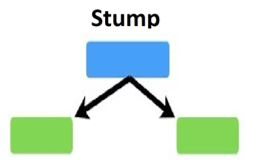# Difference between bagging and boosting

## Differences between bagging and boosting

Source

The very first thing I want to cover here is what are the differences between bagging and boosting.

To be more specific let’s take one example from each set and I say the differences between random forest and Adaboost as the random forest is bagging technique and Adaboost is a boosting technique.

Both of these come under the family of ensemble learning.

The first difference between random forest and Adaboost is random forest is a parallel learning process whereas Adaboost is a sequential learning process.

The meaning of this is in the random forest, the individual models or individual decision trees are built from the main data parallelly and independently of each other.

In the random forest, multiple trees are built from the same data parallelly and none of the trees is dependent on other trees. Hence this process is called a parallel process.

On the other hand, in the sequential process, one tree is dependent on the previous tree which means if there are multiple models implemented say ML model 1, ML model 2, ML model 3, and so on. Each of them has a process of ensembling then ML model 2 will depend on the output of ML model 1 and similarly ML model 3 will depend on the output of ML model 2.

This process in which all the models are dependent on each other or dependent on the previous model is called sequential learning.

Let me explain the second difference.

Let’s say there are multiple models fit and all these models combine to make a bigger model or a master model. In the random forest, all the models are said to have equal weights in the final model.

For example, if there are 10 models created or 10 decision trees created in a random forest then all these 10 models will have an equal vote in the final algorithm. What I mean here is all these trees are the same for the final model.

On the other hand, in Adaboost, all the trees or all the models do not have equal weights which means some of the models will have more weightage in the final model and some of the individual models will have less weightage in the final model.

The third difference between random forest and Adaboost is, in the random forest, all the individual models are one fully grown decision tree. When we say ML model 1 or decision tree model 1, in the random forest that is a fully grown decision tree. In Adaboost, the trees are not fully grown. Rather the trees are just one root and two leaves. Specifically, they are called stumps in the language of Adaboost. Stumps are nothing but
one root node and two leaf nodes.Source

So, these are basic differences between how a bagging algorithm works and how a boosting algorithm works.